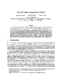## The Full Degree Spanning Tree Problem##### Files
CS-TR-3931.ps(319.19 KB)
The full degree spanning tree problem is defined as follows: given a connected graph $G=(V,E)$ find a spanning tree $T$ so as to maximize the number of vertices whose degree in $T$ is the same as in $G$ (these are called vertices of full'' degree). We show that this problem is NP-hard. We also present almost {\em optimal} approximation algorithms for it assuming $coR \neq NP$. For the case of general graphs our approximation factor is $\Theta(\sqrt{n})$. Using H{\aa}stad's result on the hardness of approximating clique, we can show that if there is a polynomial time approximation algorithm for our problem with a factor of $O(n^{\frac{1}{2}-\epsilon})$ then $coR=NP$. For the case of planar graphs, we present a polynomial time approximation scheme. Additionally, we present some experimental results comparing our algorithm to the previous heuristic used for this problem. (Also cross-referenced as UMIACS 98-47)